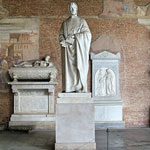# Fibonacci Day Date in the current year: November 23, 2023Fibonacci Day is observed by math geeks around the world on November 23. It was created to celebrate a renowned Italian mathematician who is widely regarded as the most talented Western mathematician of the Middle Ages.

Fibonacci, also known as Leonardo Bonacci, Leonardo Bigollo Pisano, or Leonardo of Pisa, was born circa 1170 in Pisa. His father ran a trading post in what is now Algeria, and it is there that a young Fibonacci first learned about various numeral systems and realized the advantages of the Hindu-Arabic system, which we use today thanks to him.

Fibonacci decided to introduce the system to Europe and described it in his book titled Liber Abaci, which was first published in 1202. The book described the Hindu-Arabic system, which Fibonacci called modus Indorum (method of the Indians), with its use of zero and positional notation, as well as demonstrated its practical use for a variety of applications, ranging from converting measures and weights to commercial bookkeeping.

Alongside popularization of the Hindu-Arabic system, the Fibonacci sequence is considered Fibonacci’s greatest contribution to mathematics. Although the sequence was first described by ancient Indian mathematicians, it was Fibonacci who introduced it to Western European mathematics in Liber Abaci.

The Fibonacci sequence is a sequence of numbers, known collectively as the Fibonacci numbers, in which each number is the sum of the two preceding numbers. It can start from 0 and 1, 1 and 1, and sometimes from 1 and 2. A visual representation of the Fibonacci sequence is a spiral, referred to as the Fibonacci spiral or the golden spiral.

The Fibonacci numbers appear unexpectedly often in mathematics and are strongly related to the golden ratio, when the ratio of two quantities is the same as the ratio of their sum to the larger of two quantities. The Fibonacci numbers have multiple applications in computer algorithms, technical analysis, and other fields, while the golden ratio is widely applied in art, architecture, design, and music.

The origins of Fibonacci Day are unclear, but it was probably created by someone who wanted to honor Fibonacci’s contributions to mathematics in general and the Fibonacci sequence in particular. The choice of the date makes sense since November 23 written in the mm/dd format (11/23) corresponds to the first four digits of the Fibonacci sequence.

How can you celebrate Fibonacci Day? Start with learning more about the life and work of this outstanding mathematician and the importance of the Fibonacci sequence. Share the facts you’ve learned on social media with the hashtag #FibonacciDay to let others know about this amazing holiday and encourage them to celebrate it with you.

An interesting way to celebrate Fibonacci Day is to look for the Fibonacci sequence and the golden ratio in objects around you. They can be found in nature (for example, in flowers, pine cones, ferns, pineapples, and snail shells), architecture (for example, spiral staircases), famous works of art, etc. You also can get creative and try your hand at making art featuring the Fibonacci spiral or even baking Fibonacci spiral cookies!

Remind me with

#### Category

Other Observances

#### Tags

Fibonacci Day, Fibonacci numbers, Fibonacci sequence, international observances, unofficial holidays